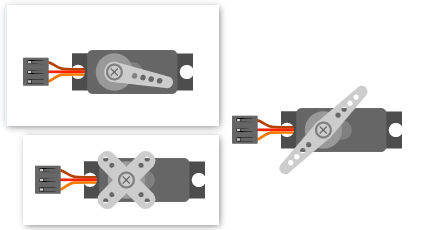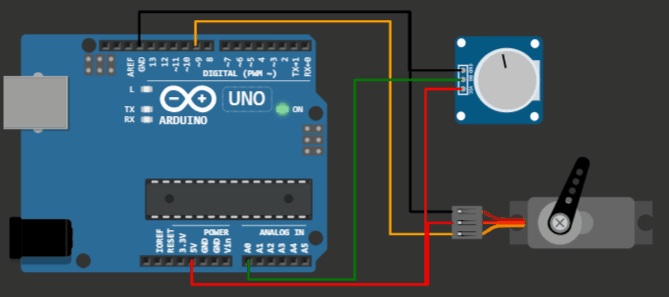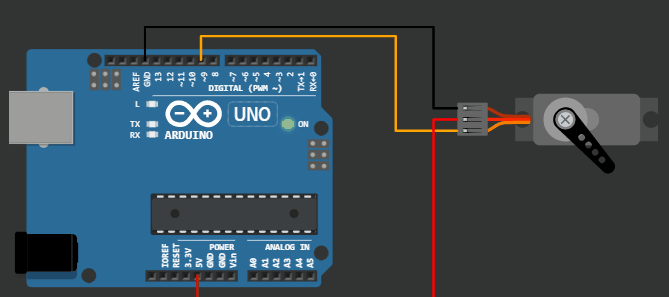This project demonstrates the code for servo motor sweep example on virtual Arduino simulator from wokwi.

Servo motor basics

Servo motors are very much user-friendly compared to other motors. The programming is easy. The software drivers are easily available.

The Servo motors consist of a small intelligent comparator inside which can be programmed using a PWM signal. The angle of rotation is controlled by the pulse width oh the PWM signal. What this means is, even if there is a slight offset in the rotation angle (due to the load or due to a small shock) it will be corrected sooner. This Servo motor feature makes it a good candidate for several applications such as robotic arms, dispensers and more.

The Servo motor element is present in the wokwi Arduino simulator. Click here to see the element propertiesServo motor element in Virtual Arduino Simulator

## Project 1: Knob code example for Servo motor on Virtual Arduino SimulatorServo motor Arduino project - knob example

```        ```/*
Controlling a servo position using a potentiometer (variable resistor)
by Michal Rinott <http://people.interaction-ivrea.it/m.rinott>

modified on 8 Nov 2013
by Scott Fitzgerald
http://www.arduino.cc/en/Tutorial/Knob
*/

#include <Servo.h>

Servo myservo;  // create servo object to control a servo

int potpin = 0;  // analog pin used to connect the potentiometer
int val;    // variable to read the value from the analog pin

void setup() {
myservo.attach(9);  // attaches the servo on pin 9 to the servo object
}

void loop() {
val = analogRead(potpin);            // reads the value of the potentiometer (value between 0 and 1023)
val = map(val, 0, 1023, 0, 180);     // scale it to use it with the servo (value between 0 and 180)
myservo.write(val);                  // sets the servo position according to the scaled value
delay(15);                           // waits for the servo to get there
}```
```

You can play with the project here on the Wokwi Arduino simulator: https://wokwi.com/arduino/libraries/Servo/Knob

## Project 2: Sweep example for Servo motorWokwi Arduino Simulator example = Servo motor 180-degree sweep

```        ```/* Sweep
by BARRAGAN <http://barraganstudio.com>
This example code is in the public domain.

modified 8 Nov 2013
by Scott Fitzgerald
http://www.arduino.cc/en/Tutorial/Sweep
*/

#include <Servo.h>

Servo myservo;  // create servo object to control a servo
// twelve servo objects can be created on most boards

int pos = 0;    // variable to store the servo position

void setup() {
myservo.attach(9);  // attaches the servo on pin 9 to the servo object
}

void loop() {
for (pos = 0; pos <= 180; pos += 1) { // goes from 0 degrees to 180 degrees
// in steps of 1 degree
myservo.write(pos);              // tell servo to go to position in variable 'pos'
delay(15);                       // waits 15ms for the servo to reach the position
}
for (pos = 180; pos >= 0; pos -= 1) { // goes from 180 degrees to 0 degrees
myservo.write(pos);              // tell servo to go to position in variable 'pos'
delay(15);                       // waits 15ms for the servo to reach the position
}
}```
```

You can tinker with the code for the project above here

More about virtual Arduino online simulator from Wokwi